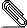# [HACKERS] Using multidimensional indexes in ordinal queries

```Hello hackers,

I would like to share some my thoughts about usage of multidimensional
indexes for queries which deal with ordinal unidimensional data types. I
think that gist indexes (especially with knngist) can produce great benefit
for complex multi-criterion queries.
Let's consider come example. I use postgresql-9.0beta1 with knngist patch.
Also I have created simple patch that allows to use knngist for ordinal
sorting in cube extension (patch is attached). The "<*>" operator was
introduced in my patch. The first operand is the cube and the second operand
is number n. If n = 2*k then the ascending ordering by k-dimension occurs.
If n = 2*k + 1 then descending ordering by k-dimension occurs. Now this
operator have a limitation and works only with nonnegative coordinate
values.
Let's create table with 3 float-point columns and fill it with 10M rows;```
```
create table test (id serial primary key, v1 double precision, v2 double
precision, v3 double precision);
insert into test (v1,v2,v3) (select random()*1000, random()*1000,
random()*1000 from generate_series(1,10000000,1));

Now, let's create 3 separate btree indexes and one gist cube index.

create index test_v1_idx on test(v1);
create index test_v2_idx on test(v2);
create index test_v3_idx on test(v3);
create index test_cube_idx on test using gist(cube(ARRAY[v1,v2,v3]));

Let's consider some complex query with filtering, ordering and limit.

test=# select * from test where v1 between 480 and 500 and v2 between 480
and 500 order by v3 limit 10;
id    |        v1        |        v2        |        v3
----------+------------------+------------------+-------------------
12283631 | 485.982828773558 | 496.795611456037 | 0.213871244341135
4936086 | 497.239370364696 | 491.878624074161 |  1.26481195911765
8963067 | 484.963194001466 | 497.094289399683 |  1.30057940259576
12435440 | 498.670902103186 | 498.667187988758 |  1.33110675960779
11667415 | 494.398592971265 | 497.440234292299 |  1.44533207640052
8530558 |  482.85893118009 | 496.267838869244 |  1.48530444130301
4004942 | 483.679085504264 | 489.547223784029 |  1.57393841072917
14897796 |  491.37338064611 | 487.475242000073 |  1.81775307282805
4105759 | 489.506138022989 |  486.91446846351 |  1.94038823246956
12895656 | 499.508572742343 | 487.065799534321 |  2.34963605180383
(10 rows)

test=# explain analyze select * from test where v1 between 480 and 500 and
v2 between 480 and 500 order by v3 limit 10;

QUERY PLAN

-------------------------------------------------------------------------------------------------------------------------------------------------------------------
Limit  (cost=22786.73..22786.75 rows=10 width=28) (actual
time=3242.135..3242.162 rows=10 loops=1)
->  Sort  (cost=22786.73..22797.59 rows=4345 width=28) (actual
time=3242.131..3242.144 rows=10 loops=1)
Sort Key: v3
Sort Method:  top-N heapsort  Memory: 25kB
->  Bitmap Heap Scan on test  (cost=8755.91..22692.83 rows=4345
width=28) (actual time=1281.030..3234.934 rows=4027 loops=1)
Recheck Cond: ((v1 >= 480::double precision) AND (v1 <=
500::double precision) AND (v2 >= 480::double precision) AND (v2 <=
500::double precision))
->  BitmapAnd  (cost=8755.91..8755.91 rows=4345 width=0)
(actual time=1280.783..1280.783 rows=0 loops=1)
->  Bitmap Index Scan on test_v1_idx
(cost=0.00..4243.12 rows=202177 width=0) (actual time=644.702..644.702
rows=200715 loops=1)
Index Cond: ((v1 >= 480::double precision) AND
(v1 <= 500::double precision))
->  Bitmap Index Scan on test_v2_idx
(cost=0.00..4510.37 rows=214902 width=0) (actual time=630.085..630.085
rows=200200 loops=1)
Index Cond: ((v2 >= 480::double precision) AND
(v2 <= 500::double precision))
Total runtime: 3242.253 ms
(12 rows)

This query can be rewritten in order to let planner use gist cube index.

test=# select * from test where cube(array[v1,v2,v3]) <@
cube(array[480,480,'-Infinity'::float],array[500,500,'+Infinity'::float])
order by cube(array[v1,v2,v3]) <*> 4 limit 10;
id    |        v1        |        v2        |        v3
----------+------------------+------------------+-------------------
12283631 | 485.982828773558 | 496.795611456037 | 0.213871244341135
4936086 | 497.239370364696 | 491.878624074161 |  1.26481195911765
8963067 | 484.963194001466 | 497.094289399683 |  1.30057940259576
12435440 | 498.670902103186 | 498.667187988758 |  1.33110675960779
11667415 | 494.398592971265 | 497.440234292299 |  1.44533207640052
8530558 |  482.85893118009 | 496.267838869244 |  1.48530444130301
4004942 | 483.679085504264 | 489.547223784029 |  1.57393841072917
14897796 |  491.37338064611 | 487.475242000073 |  1.81775307282805
4105759 | 489.506138022989 |  486.91446846351 |  1.94038823246956
12895656 | 499.508572742343 | 487.065799534321 |  2.34963605180383
(10 rows)

test=# explain analyze select * from test where cube(array[v1,v2,v3]) <@
cube(array[480,480,'-Infinity'::float],array[500,500,'+Infinity'::float])
order by cube(array[v1,v2,v3]) <*> 4 limit 10;
QUERY PLAN

-------------------------------------------------------------------------------------------------------------------------------------
Limit  (cost=0.00..39.10 rows=10 width=28) (actual time=5.186..20.047
rows=10 loops=1)
->  Index Scan using test_cube_idx on test  (cost=0.00..39100.88
rows=10000 width=28) (actual time=5.177..20.012 rows=10 loops=1)
Index Cond: (cube(ARRAY[v1, v2, v3]) <@ '(480, 480, -inf),(500,
500, inf)'::cube)
Sort Cond: (cube(ARRAY[v1, v2, v3]) <*> 4)
Total runtime: 20.145 ms
(5 rows)

So, the result is the same, but query runs about 150 time faster.
I think that usage of multidimensional index on ordinal data types can
produce following benefits:
1) It will be possible to create one multidimensional index instead of many
of unidimensional ones. (Of course, it will be slower on simple queries).
2) On some complex queries (like noted above) it can produce
great performance benefit.

And now there is my question. How huge changes in planner is needed to make
planner choose multidimensional index freely without rewriting query in
special manner? In my example I replaced "v1 between 480 and 500 and v2
between 480 and 500" by "cube(array[v1,v2,v3]) <@
cube(array[480,480,'-Infinity'::float],array[500,500,'+Infinity'::float])"
and "order by v3" by "order by cube(array[v1,v2,v3])", but it will be a
great option if planner could consider plan with gist cube index for
original query.

With best regards,
Alexander Korotkov.
```cube.gz
Description: GNU Zip compressed data

```--
Sent via pgsql-hackers mailing list (pgsql-hackers@postgresql.org)
To make changes to your subscription:
http://www.postgresql.org/mailpref/pgsql-hackers
```